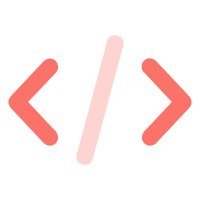#Ahonn's Blog

## apply, call 与 bind 的区别

2016年03月20日预计阅读需要 4 分钟

## apply() 与 call()

`apply()` 方法接收两个参数，一个是在其中运行函数的作用域，函数中的 `this` 的值，另一个是参数数组。 而 `call()` 方法的第一个参数跟 `apply()` 相同，不同的是除了第一个参数，后面的其他参数将会直接传递给函数。

``````function sum(num1, num2) {
return num1 + num2;
}

// 使用 apply()
function applySum(num1, num2) {
return sum.apply(this, arguments);
}

// 使用 call()
function callSum(num1, num2) {
return sum.call(this, num1, num2);
}

alert(applySum(1, 2)); // 3
alert(callSum(1, 2)); // 3``````

``````name = "unknown";
var person = {
name = "ahonn";
}

function sayName() {
console.log(this.name);
}

sayName() // unknown
// 函数中的 this 为全局环境
sayName.apply(this); // unknown
// 函数中的 this 指向了 person
sayName.apply(person) // ahonn``````

## bind()

`bind()` 方法与 `apply()``call()` 相似，同样是可以改变函数内 this 的指向。但与 `apply()`, `call()` 不同的是，`bind()` 方法会创建一个新函数，称为绑定函数，当调用绑定函数时，函数会以创建时的第一个参数 this 作为函数运行的作用域。

``````name = "unknown";
var person = {
name = "ahonn";
}

function sayName() {
console.log(this.name);
}

var sayAhonnName = sayName.bind(person);

// 函数中的 this 指向 person
sayAhonnName() // ahonn``````

``````var foo = {
bar: 1,
eventBind: function() {
var _this = this;
\$('.someClass').on('click', function(event) {
console.log(_this.bar);
});
}
}``````

``````var foo = {
bar : 1,
eventBind: function(){
\$('.someClass').on('click',function(event) {
console.log(this.bar);
}.bind(this));
}
}``````